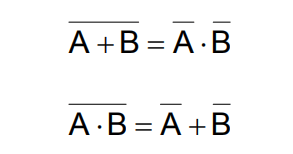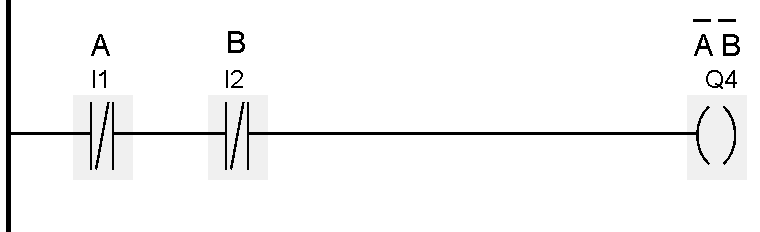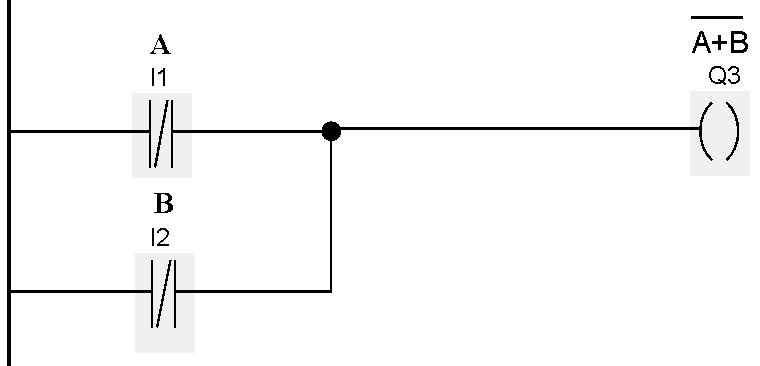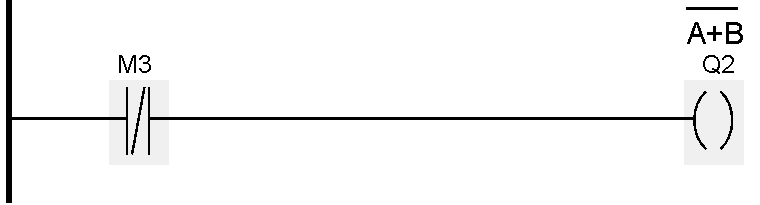Home » DeMorgan’s Theorems using Ladder Diagram
PLC Tutorials

# DeMorgan’s Theorems using Ladder Diagram

DeMorgan theorem is used in digital electronics. Explain the De Morgan theorem using PLC ladder language.

DeMorgan’s Theorems are two additional simplification techniques that can be used to simplify Boolean expressions. Again, the simpler the Boolean expression the simpler the resulting the Boolean expression, the simpler the resulting logic## DeMorgan’s Theorems### Explanation

De Morgan theorem provides equality between NAND gate and negative OR gate and the equality between the NOR gate and the negative AND gate.

For example, take two variables A and B. The theorem is mathematical stated as, AB=A+B. The complement of the two variables is equal to the OR of complements of individual variables.

Second theorem is stated as: The complement of two variables ORed is equal to the AND of the complements of the individual variables.

### List of Inputs/Outputs

• I1 :- A
• I2 :- B

• Q1 :- OP 1
• Q2 :- OP 2
• Q3 :- OP 3
• Q4 :- OP 4

#### Memory coil

• M2 :- Memory coil for AND operation
M3 :- Memory coil for OR operation

#### NETWORK 1 :-

AND operation is used as shown in below figure. Result will be stored in M2 coil.#### NETWORK 2 :-

Inverse logic is used for DeMorgan first law as per below figure. It will generate inverse output (Q1).#### NETWORK 3 :-

Two NC contact of variable A and variable B are connected in OR connection. Result will be Q3.Note :- LOGIC OPERATION OF Q1 AND Q3 ARE EQUAL AS PER LAW.

#### NETWORK 4 :-

Combination of two variables. Result will be stored in M3 variable.#### NETWORK 5 :-

Inverse logic is used. Output Q2 will work inversely as per the law.#### NETWORK 6 :-

NC contacts of two variables are used in this network in AND gate.NOTE:-THE LOGIC OPERATION OF Q2 AND Q4 IS EQUAL AS PER LAW.

Note :- The above application may be different from actual application. This example is only for explanation and educational purpose only. We can implement this logic in other PLC. This is the simple concept of De Morgan theorems using ladder logic, we can use this concept in other examples.

All parameters and graphical representations considered in this example are for explanation purpose only, parameters or representation may be different in actual applications. All interlocks are not considered in the application.

#### Articles You May Like :

PLC Troubleshooting

PLC Conveyor Program

Motor Logic using PLC

PLC Practical Tests

Switches, Routers, Firewalls

#### Automatic Empty Bottle detection using PLC Logic

This website uses cookies to improve your experience. We'll assume you're ok with this, but you can opt-out if you wish. Accept Read More

WordPress Image Lightbox

Send this to a friend